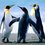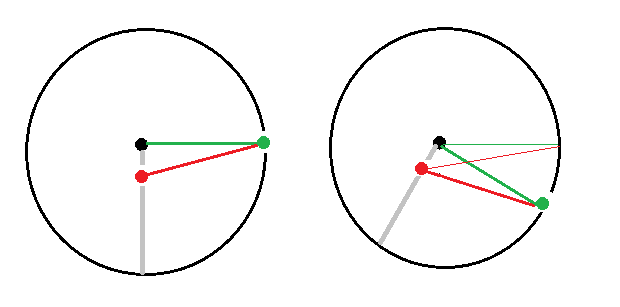# Classical mechanics problem

There is a uniform disc of mass $m$ and radius $r$ placed on a rough horizontal ground, A rod of same mass and length $r$ is pivoted to the centre of the disc at its end and is initially vertical, the rod being above the centre(unstable eq)...now the rod is disturbed slightly. Find the acceleration of the disc when the rod gas rotated by an angle $\theta$. The floor is rough enough to prevent slipping.Note by Kudo Shinichi
2 years, 7 months ago

This discussion board is a place to discuss our Daily Challenges and the math and science related to those challenges. Explanations are more than just a solution — they should explain the steps and thinking strategies that you used to obtain the solution. Comments should further the discussion of math and science.

When posting on Brilliant:

• Use the emojis to react to an explanation, whether you're congratulating a job well done , or just really confused .
• Ask specific questions about the challenge or the steps in somebody's explanation. Well-posed questions can add a lot to the discussion, but posting "I don't understand!" doesn't help anyone.
• Try to contribute something new to the discussion, whether it is an extension, generalization or other idea related to the challenge.

MarkdownAppears as
*italics* or _italics_ italics
**bold** or __bold__ bold
- bulleted- list
• bulleted
• list
1. numbered2. list
1. numbered
2. list
Note: you must add a full line of space before and after lists for them to show up correctly
paragraph 1paragraph 2

paragraph 1

paragraph 2

[example link](https://brilliant.org)example link
> This is a quote
This is a quote
    # I indented these lines
# 4 spaces, and now they show
# up as a code block.

print "hello world"
# I indented these lines
# 4 spaces, and now they show
# up as a code block.

print "hello world"
MathAppears as
Remember to wrap math in $$ ... $$ or $ ... $ to ensure proper formatting.
2 \times 3 $2 \times 3$
2^{34} $2^{34}$
a_{i-1} $a_{i-1}$
\frac{2}{3} $\frac{2}{3}$
\sqrt{2} $\sqrt{2}$
\sum_{i=1}^3 $\sum_{i=1}^3$
\sin \theta $\sin \theta$
\boxed{123} $\boxed{123}$

Sort by:

Can anyone give a try to this question ?

- 2 years, 7 months ago

Would you mind if I posted a problem on this one? I will also provide a solution there

- 2 years, 7 months ago

Yeah it would be very great sir

- 2 years, 7 months ago

Also sir I have two more problems , can u pls try those also ??

- 2 years, 7 months ago

Sure, I can look at those too

- 2 years, 7 months ago

{https://artofproblemsolving.com/community/c164h1757258_thermodynamics} question is in this link sir

- 2 years, 7 months ago

Also can u prove it sir in case of a situation when in a ring a rod (same mass ) is attached with its one end attached to one point on ring , and it does pure rolling . And length of rod is equal to radius , that the angular velocity w by which the the ring rotates about its centre is same with respect to net COM which is at R/4 above the COM of ring ?? Some say that as(rod) its welded so it will have same w ,but i can't understand , can u prove it sir pls ??

- 2 years, 7 months ago

The black dot is the center of the circle. The red dot is the center of mass. The green dot is a point on the circle. To track the rotation of the green point about the other two points, first draw vectors from the black and red points to the green point. Then rotate the circle to simulate a bit of rolling. Draw new vectors as before, but keep the old vectors as well (for reference). You can see that the angle difference between the two red vectors and the two green vectors is the same. This could probably be made rigorous, but I'll just leave it at this.- 2 years, 7 months ago

Sir I can't see how those angles same relate with the w to be same ? Can u explain a bit more sir ??

- 2 years, 7 months ago

If one point is rotating around another point, you can measure the rate of rotation by drawing a vectors between the two points at different times, taking the angle difference, and dividing by the elapsed time. That's what I'm doing here, and I'm doing it for the green point with respect to the black point, and for the green point with respect to the red point. Over some period of time, the elapsed angle between the green vectors is the same as the elapsed angle between the red vectors. Hence, the rate of rotation is the same (at least approximately). You would need some more rigorous analysis to really prove it.

- 2 years, 7 months ago

Sir I got that , but any rigorous proof u know about in any site ?? In brilliant ?? Or any book page number ?? Sir

- 2 years, 7 months ago

- 2 years, 7 months ago

Thermodynamics is really not a good subject for me, so I won't generally answer those. But, in this case, I will start with the ideal gas equation:

$P V = n R T \\ n = \frac{P V}{R T}$

Assume that the individual chambers are kept at their original temperatures even after the valve opens (this may or may not be allowed). After the valve opens, both sides are at the same pressure. The total number of moles of gas remain the same:

$\frac{P_{P0} V_P}{R \, T_P} + \frac{P_{Q0} \, 4 V_P}{R \, T_Q} = \frac{P_{F} V_P}{R \, T_P} + \frac{P_{F} \, 4 V_P}{R \, T_Q} \\ P_F = \frac{\frac{P_{P0} V_P}{R \, T_P} + \frac{P_{Q0} \, 4 V_P}{R \, T_Q}}{\frac{ V_P}{R \, T_P} + \frac{ \, 4 V_P}{R \, T_Q}} \\ P_F = \frac{\frac{P_{P0}}{ T_P} + \frac{P_{Q0} \, 4}{ T_Q}}{\frac{ 1}{T_P} + \frac{ 4 }{ T_Q}} \\ = \frac{\frac{5 \times 10^5}{ 300} + \frac{2 \times 10^5 \, 4}{ 400}}{\frac{ 1}{300} + \frac{ 4 }{ 400}} = 2.75 \times 10^5$

If this disagrees with the expected answer, I suppose it is because the assumption that the chambers are kept at their original temperatures is incorrect. But I don't know how to determine the final temperature otherwise. Maybe you can think of a way.

- 2 years, 7 months ago

Sir can't we get final temperature by conserving internal energy ??

- 2 years, 7 months ago

Perhaps so. How would you do it? Like I said, thermo is not a good subject for me.

- 2 years, 7 months ago

n1CvT1 + n2CvT2 = (n1+n2)CvT sir , just by this I can't get any of the options . I am getting 2.6 by this

- 2 years, 7 months ago

you must be from Physics Stack exchange?  is used there

- 2 years, 5 months ago

Nope bro just habit of typing that

- 2 years, 5 months ago

I got the answer as $a = \dfrac{12mr^2}{5}$ but don't whether it is correct or not. @Steven Chase what answer did you get ?

- 2 years, 7 months ago

Answer is likely very complex as said by my friend . between can anyone try this question and tell me why hinge will cause horizontal velocity component to be zero?? And does hinge always cause the horizontal component velocity to get zero ?? @Steven Chase sir can u check {https://goiit.com/uploads/default/original/3X/e/8/e8c02dac9e872d37113e90bd480c1119829ba456.jpg}

- 2 years, 7 months ago

@Steven Chase sir pls aware me when u post the problem sir , also pls check the question I posted before pls sir .

- 2 years, 7 months ago

It's up now. If nobody posts a solution within the next day, I'll post one.
https://brilliant.org/problems/rod-disk-combo-dynamics/

- 2 years, 7 months ago

Very very thx sir

- 2 years, 7 months ago

@Steven Chase Sir can u pls check this one too :, {https://goiit.com/uploads/default/original/3X/e/8/e8c02dac9e872d37113e90bd480c1119829ba456.jpg}

- 2 years, 7 months ago

with what is it colliding ? with ground ?

- 2 years, 7 months ago

Yeah ground bro . and me 12th

- 2 years, 7 months ago

For this one, we can figure out the angular velocity of the rod right before the collision by converting the change in gravitational potential energy into rotational kinetic energy:

$m g \frac{1}{2} = \frac{1}{2} (\frac{1}{3} m L^2) \omega^2 \\ g = \frac{1}{3} L^2 \omega^2 \\ \omega = \sqrt{\frac{3 g}{L^2}} = \sqrt{\frac{3 g}{4}} = \frac{\sqrt{3g}}{2} = \frac{4 \sqrt{3g}}{8}$

If we assume that $e = 1$ means that the kinetic energy of the rod is the same before and after the collision, this is also the angular speed after the collision.

- 2 years, 7 months ago

But @Steven Chase sir , the answer given for this problem is 3root3/8 option A.. My doubt is can we say the horizontal component of velocity at the moment of the strike , will get reduced to zero , due to hinge reaction sir ?? , then we can get the option A easily. And does hinge always cause the horizontal component velocity to get zero ??

- 2 years, 7 months ago

At that particular angle, the horizontal velocity of the rod end cannot be zero without the vertical velocity of the rod end also being zero. Suppose $\vec{u}$ is a unit vector perpendicular to the rod.

$v_x = L \omega \, u_x \\ v_y = L \omega \, u_y \\ u_x \neq 0 \\ u_y \neq 0$

If the angular speed is non-zero, and the rod is not purely horizontal, the x-component of the rod end's velocity cannot be zero. Have I misunderstood what you said?

- 2 years, 7 months ago

Sir I mean before striking it will have both(vertical and horizontal) ,but after striking can't hinge reaction cause the horizontal component to get reduced to zero ?? Sir also can u pls try this one too , I dijt know any method to find accelaration of pulley P1 , pls suggest : https://artofproblemsolving.com/community/c164h1758204nlmproblem

- 2 years, 7 months ago

I think it is impossible for only one component to be zero, if the rod isn't either horizontal or vertical.

- 2 years, 7 months ago

Ok thx sir likely answer key us wrong given . pls try the problem I posted sir above

- 2 years, 7 months ago

https://i.imgur.com/EVydoX0.jpg pls see this , should nt the answer be b ???

- 2 years, 7 months ago

I agree with you. Because $v_1$ and $v_2$ are stated as being relative to the the large block, their magnitudes should be equal. If instead, $v_1$ and $v_2$ were given as being relative to the observer, the relationship would be $v_1 - v_0 = v_2$.

- 2 years, 7 months ago

Yeah thx a lot sir

- 2 years, 7 months ago

@Azimuddin Sheikh, which standard you are, 11 or 12 ? And also, in which branch of fitjee are you studying ?

- 2 years, 7 months ago

I have posted a solution to yesterday's problem

- 2 years, 7 months ago

Thx a lot sir !!!!!

- 2 years, 7 months ago

My solution resulted in a coupled set of differential equations, with no particularly simple solution. I'm going to derive it again to check my work, and then post a problem on it.

- 2 years, 7 months ago